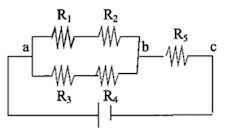Problem: A combination of resistors, shown in the accompanying figure, is attached to a 10 V battery. Assume R1 = R2 = 5Ω, R3 = R4 = 15Ω, and R5 = 20Ω.Find the equivalent resistor.Find the current through the resistor R1Find the current through the resistor R3Find the voltage drop across the resistor R2.Find the voltage drop across the resistor R5.

FREE Expert Solution
93% (258 ratings)
Problem Details

A combination of resistors, shown in the accompanying figure, is attached to a 10 V battery. Assume R1 = R2 = 5Ω, R3 = R4 = 15Ω, and R5 = 20Ω.

1. Find the equivalent resistor.
2. Find the current through the resistor R1
3. Find the current through the resistor R3
4. Find the voltage drop across the resistor R2.
5. Find the voltage drop across the resistor R5